# Tangents and Secants to a Circle solutions class 10 SSC

Mathematics class 10 solutions for Tangents and Secants to a Circle exercises 9,1, 9.2 and 9.3 are given.

These solutions are very easy to understand.

First you study the lesson Tangents and Secands to a Circle in the text book very well.

Observe the example problems and solutions in the text book.

You can see text book solutions for class 10

2. Sets

9. Tangents and Secants to a Circle

10. Mensuration

11. Trigonometry

13. Probability

14. Statistics

# Maths solutions for class 10 Tangents and secants to a circle SSC

Chapter 9 ssc solutions class 10

Exercise 9.1 – Tangents and Secants to a Circle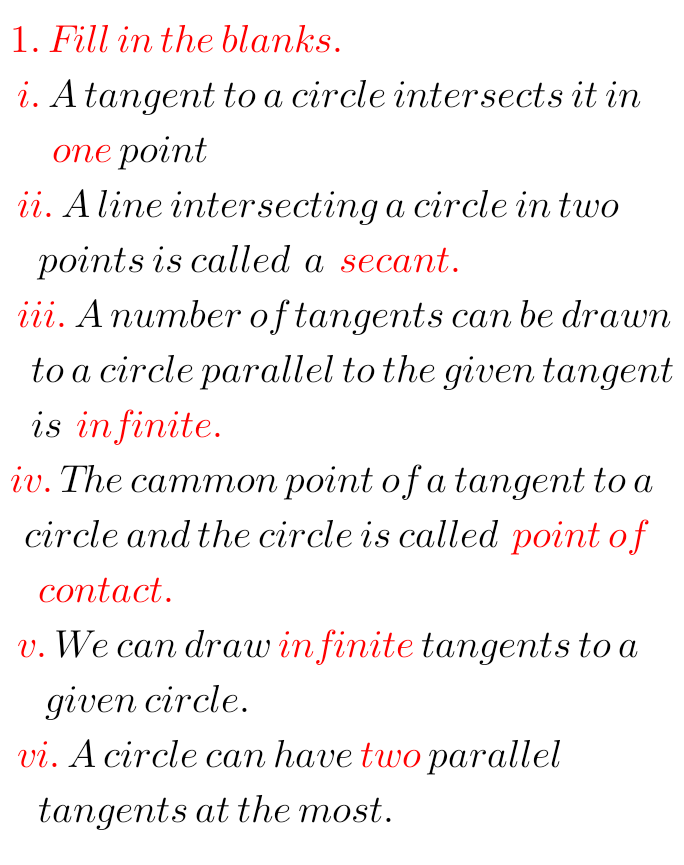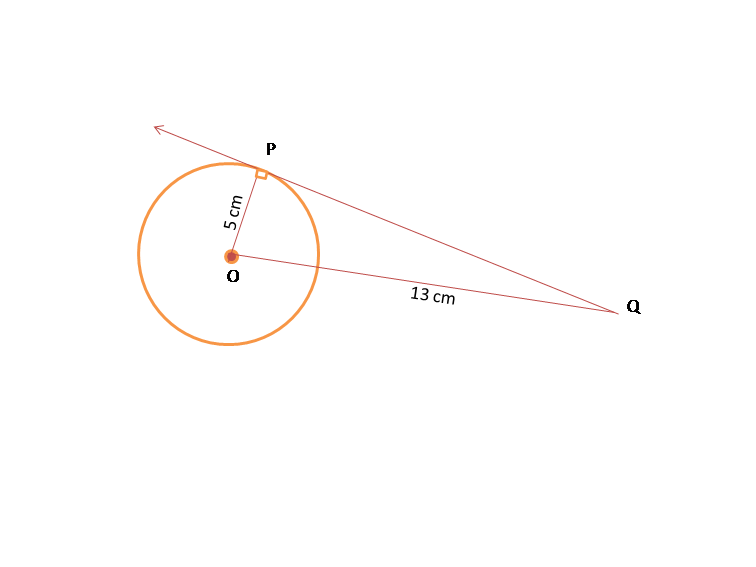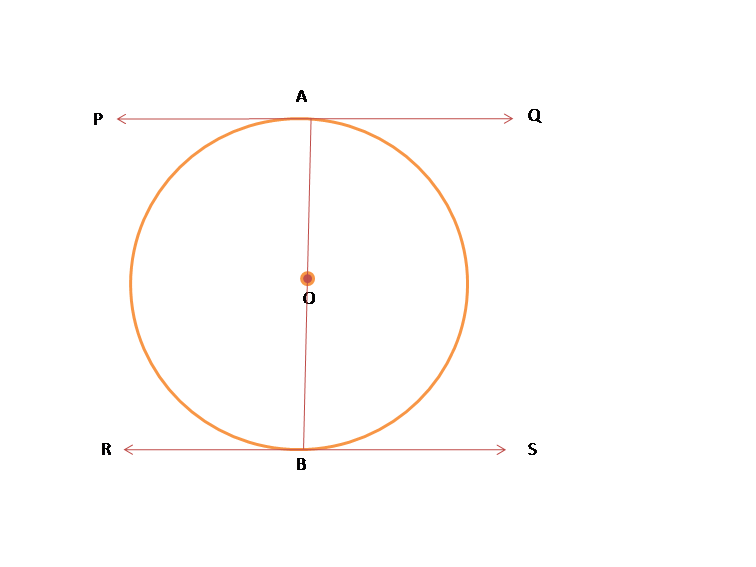## Tenth maths solutions for tangents and secants to a circle SSC

Exercise 9.2 class 10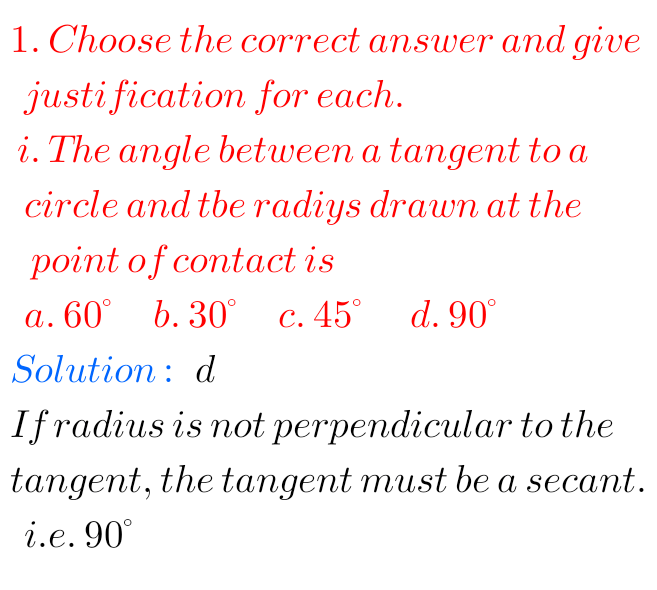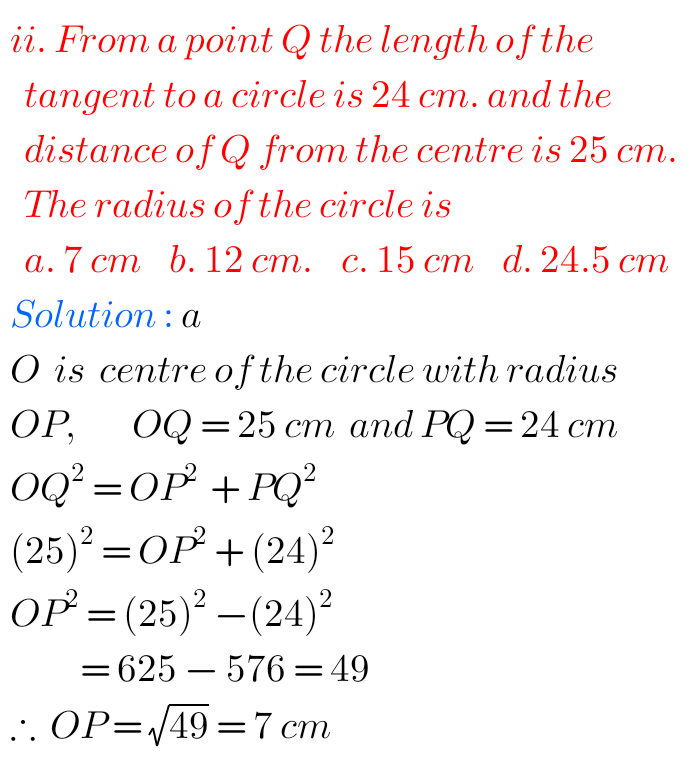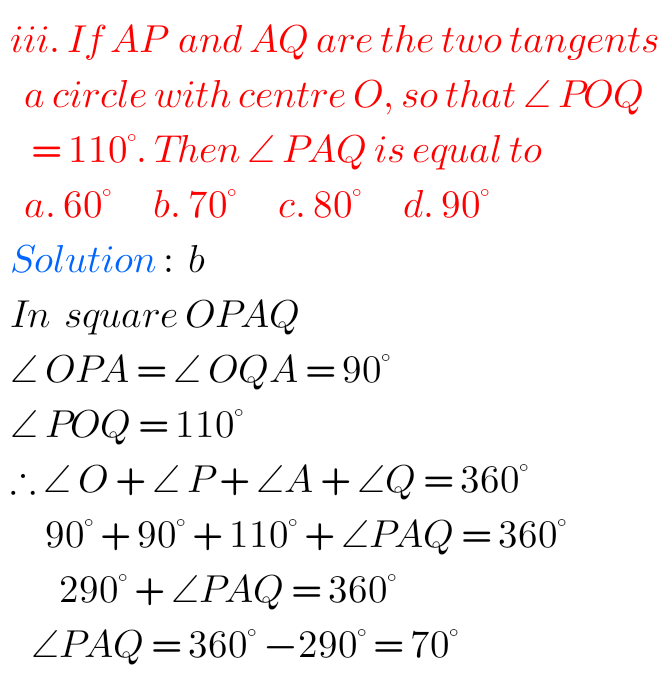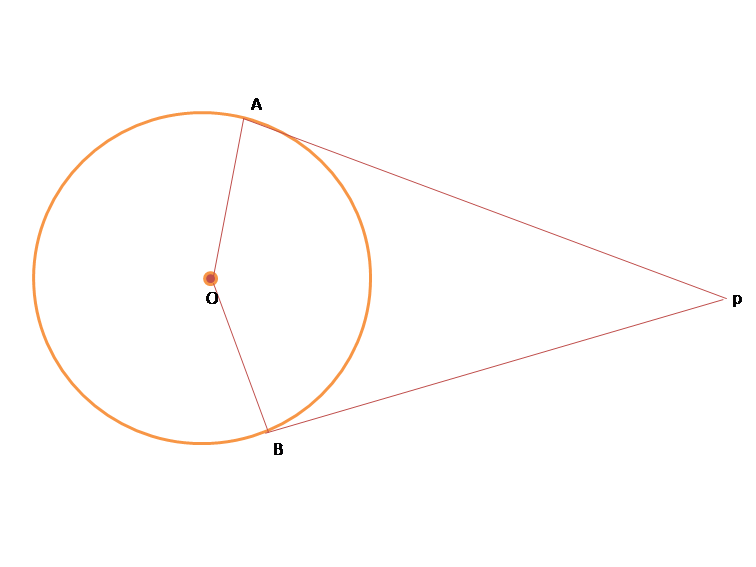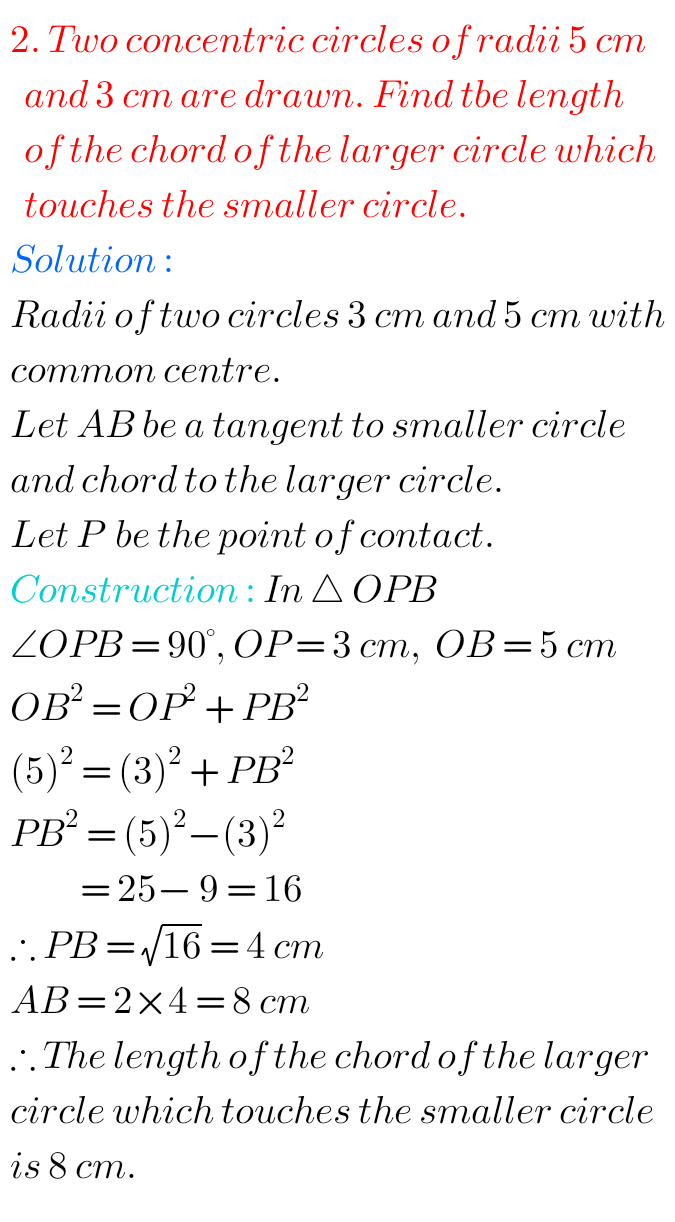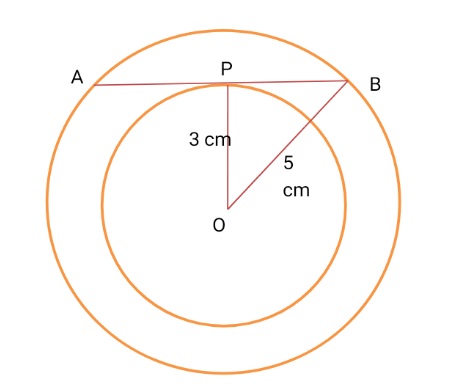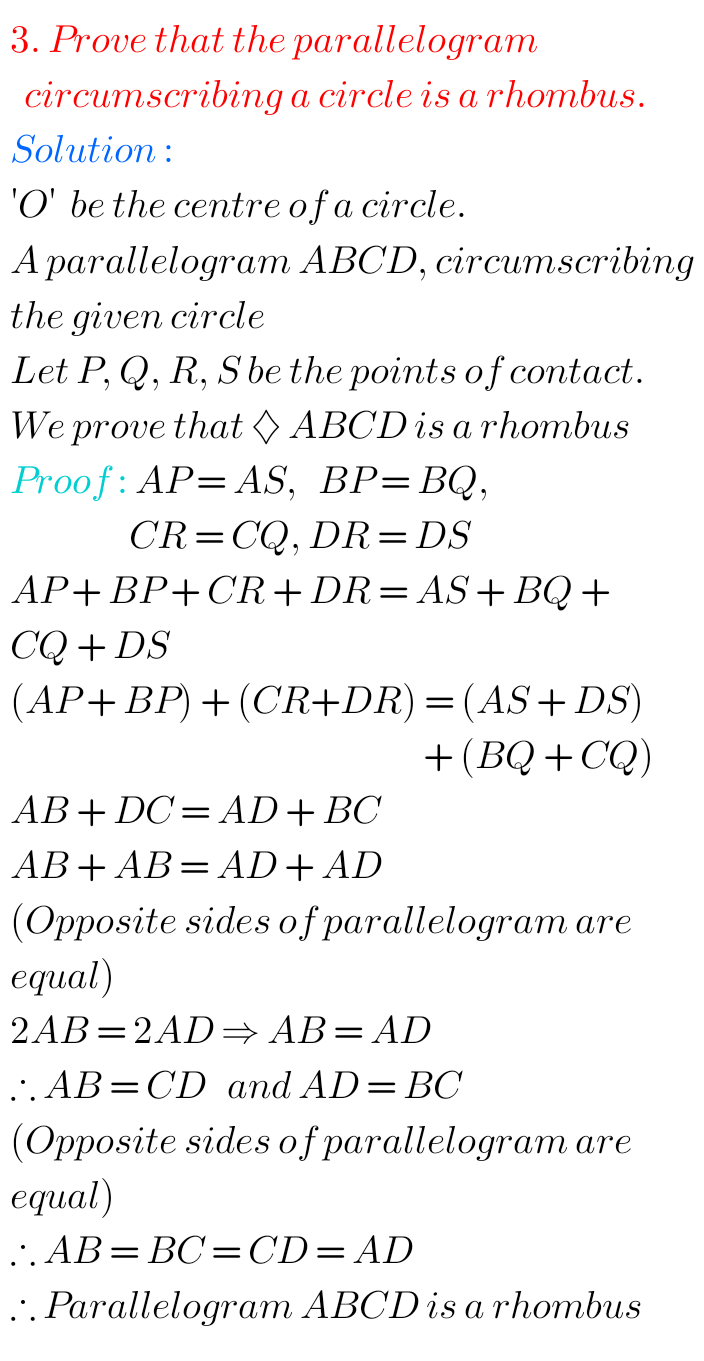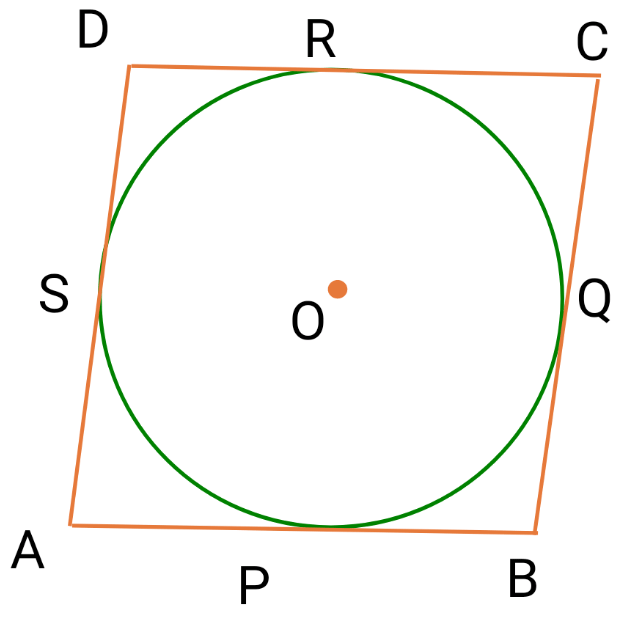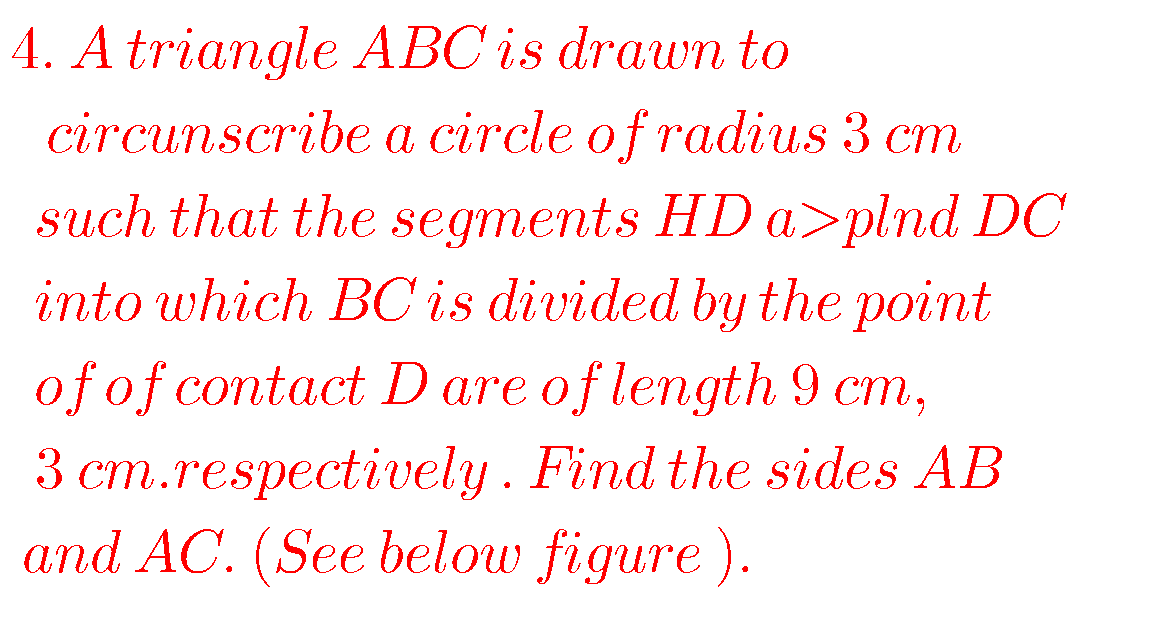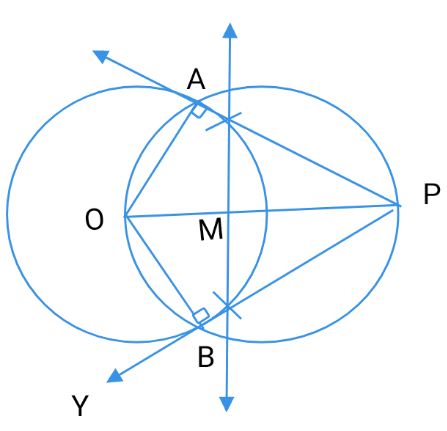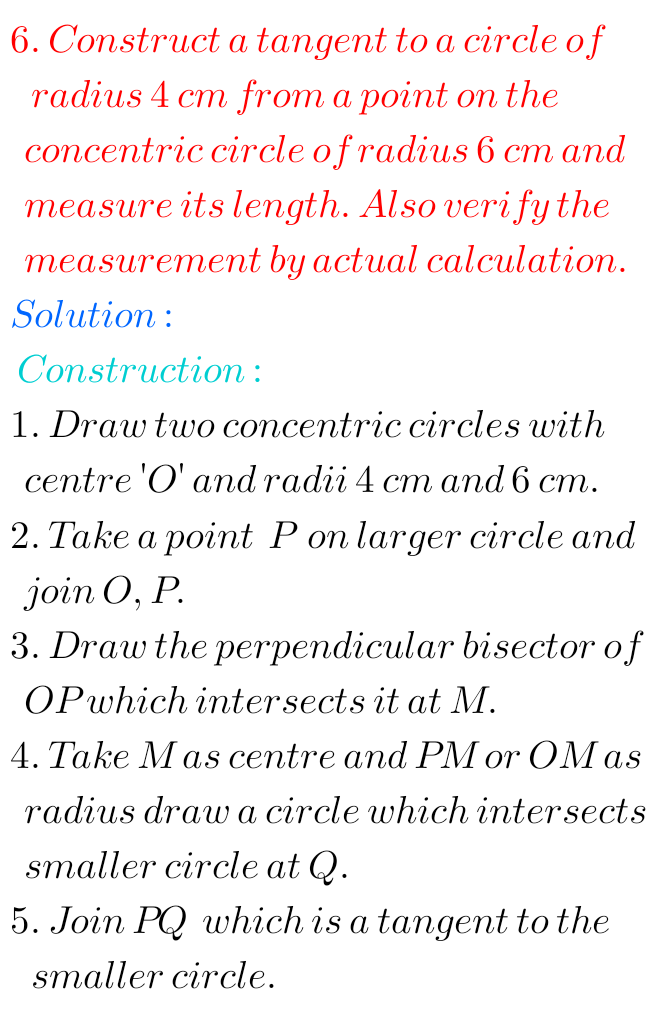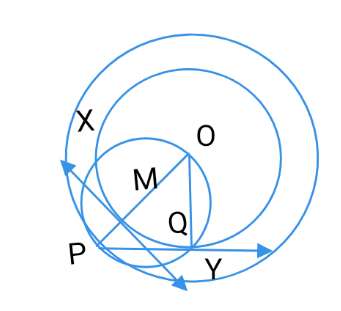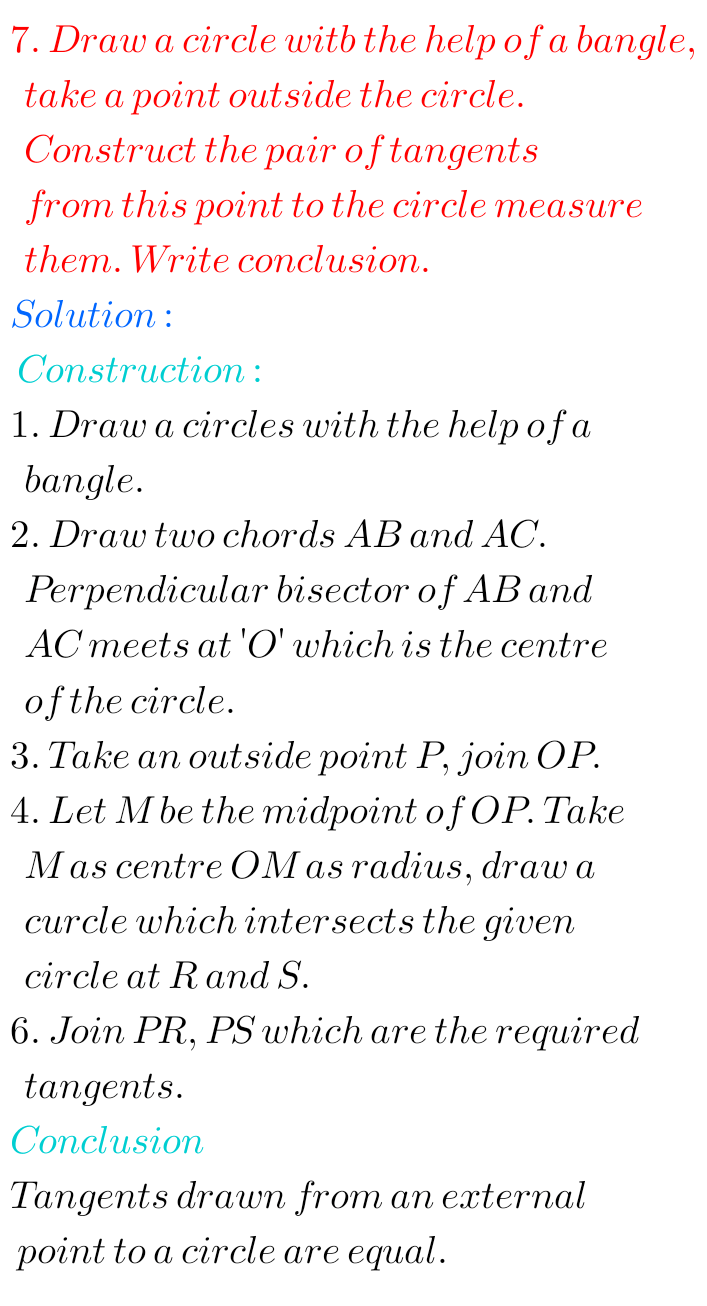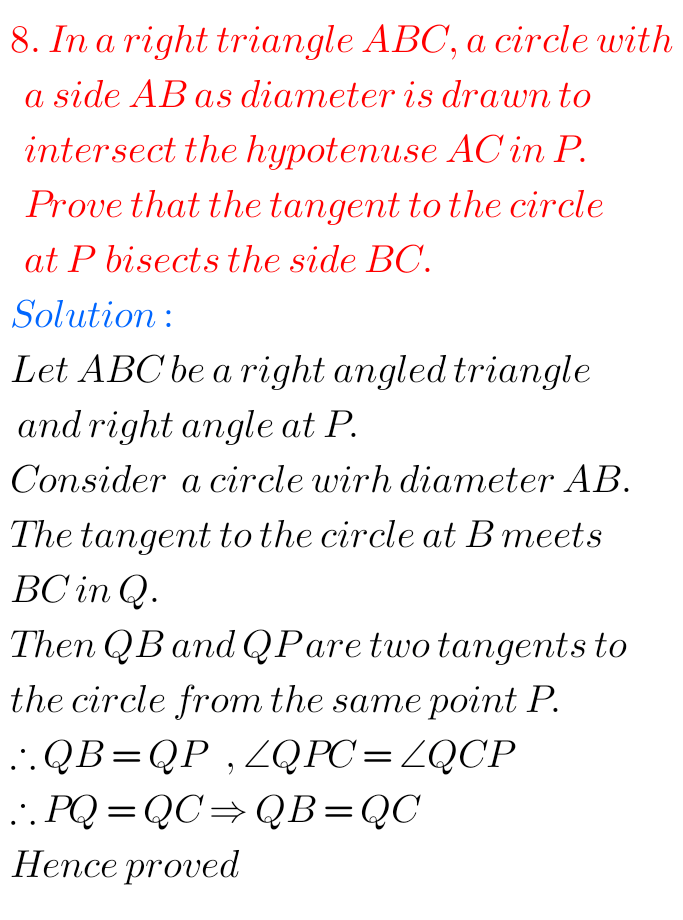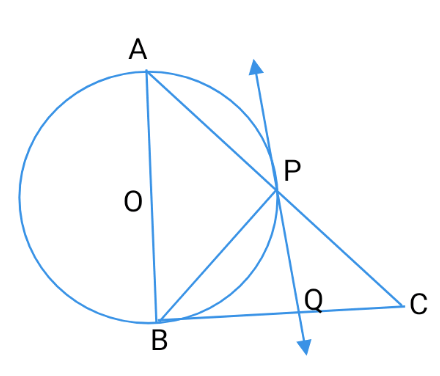### Solutions for tangents and secants to a circle SSC AP and TS

Exercise 9.3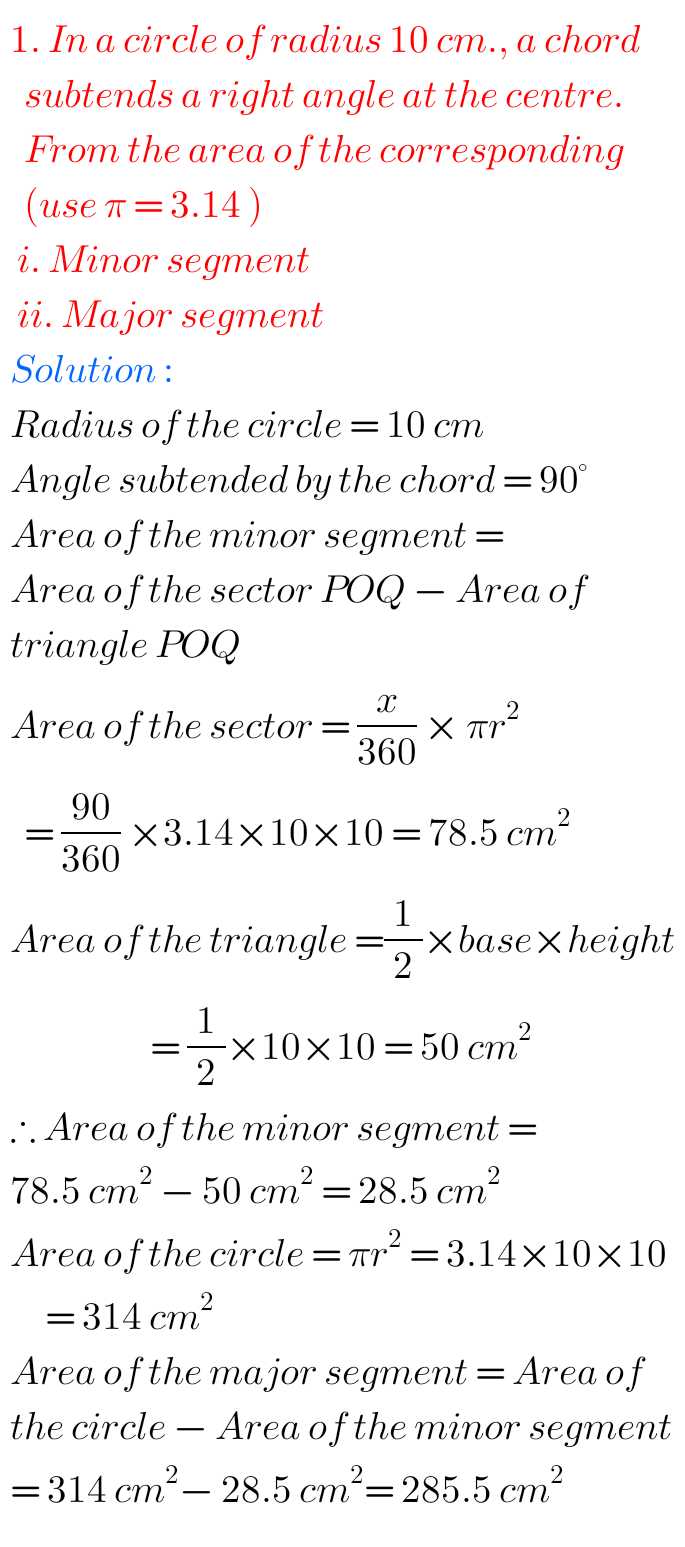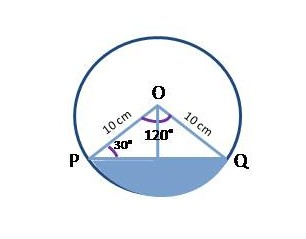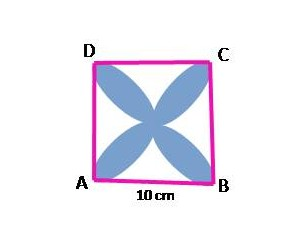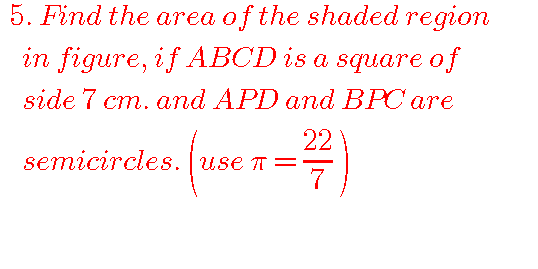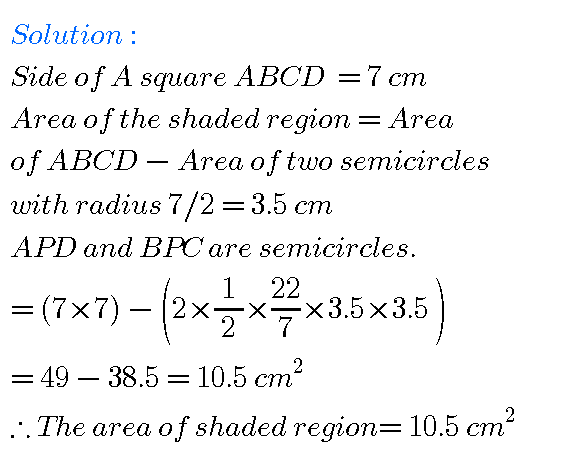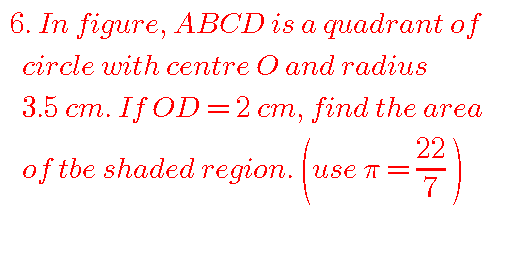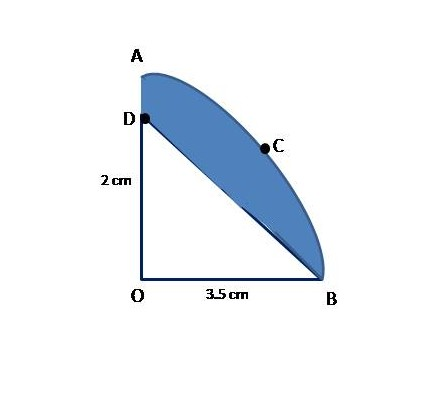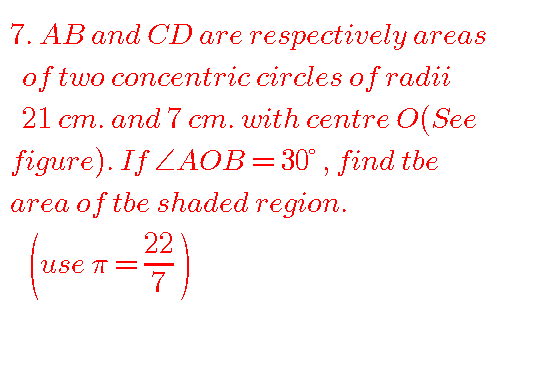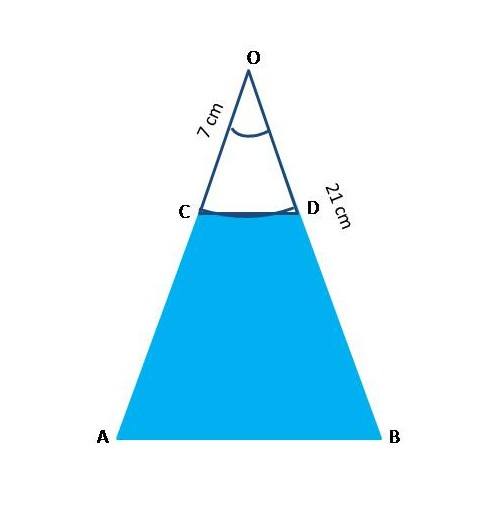Optional ExerciseFigure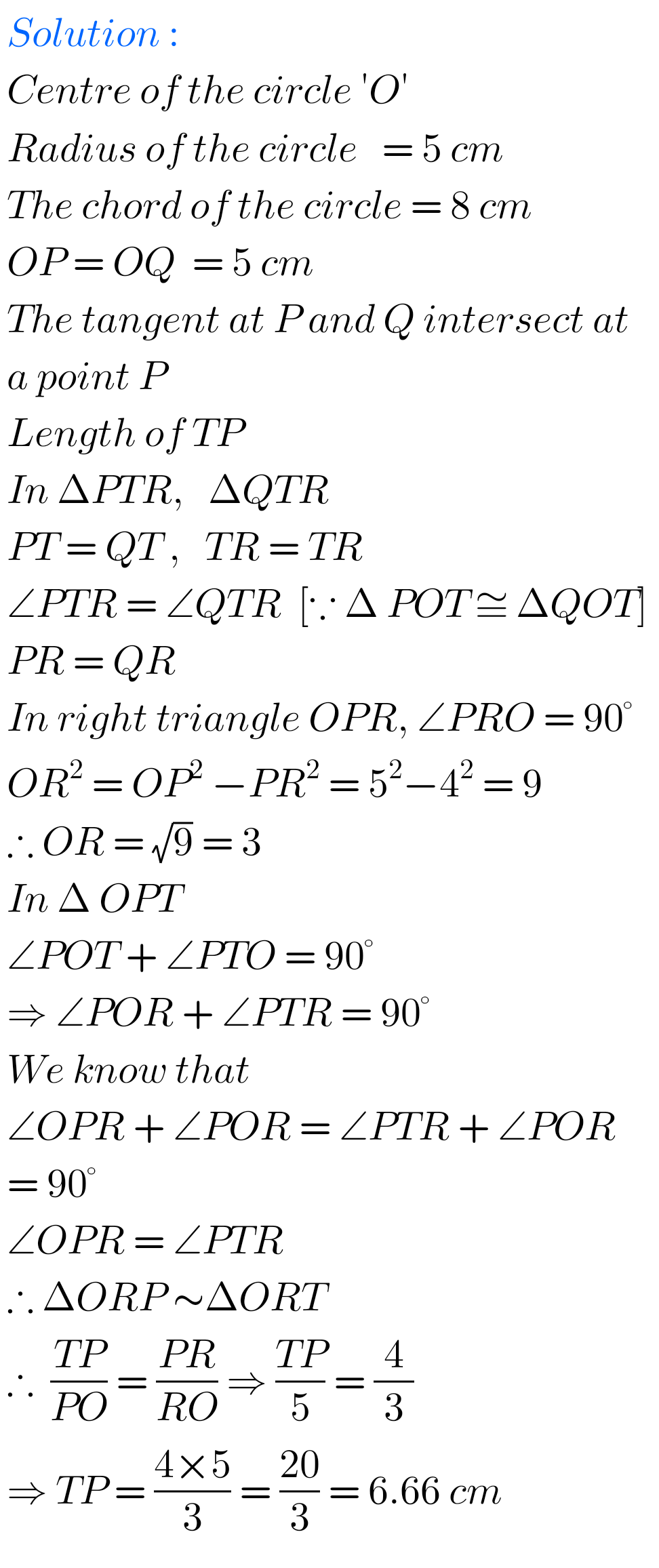M

Note : Observe the solutions and try them in your own method.

You can also see

Inter Maths 1A textbook solutions

Inter Maths 1B textbook solutions

Inter Maths IIA textbook solutions

Inter Maths IIB textbook solutions